Courses

# Bessel's Special Function (Part - 2) - Mathematical Methods of Physics, UGC - NET Physics Physics Notes | EduRev

## Physics for IIT JAM, UGC - NET, CSIR NET

Created by: Akhilesh Thakur

## Physics : Bessel's Special Function (Part - 2) - Mathematical Methods of Physics, UGC - NET Physics Physics Notes | EduRev

The document Bessel's Special Function (Part - 2) - Mathematical Methods of Physics, UGC - NET Physics Physics Notes | EduRev is a part of the Physics Course Physics for IIT JAM, UGC - NET, CSIR NET.
All you need of Physics at this link: Physics

Bessel Functions of Nonintegral Order

These different approaches are not exactly equivalent. The generating function approach is very convenient for deriving two recurrence relations, Bessel’s differential equation, integral representations, addition theorems, and upper and lower bounds. However, you will probably have noticed that the generating function deﬁned only Bessel functions of integral order, J0 ,J1 ,J2 , and so on. This is a limitation of the generating function approach that can be avoided by using the contour integral instead, thus leading to foregoing approach (3). But the Bessel function of the ﬁrst kind, Jν (x ), may easily be deﬁned for nonintegral ν by using the series (Eq. (11.5)) as a new deﬁnition.
The recurrence relations may be veriﬁed by substituting in the series form of Jν (x ). From these relations Bessel’s equation follows. In fact, if ν is not an integer, there is actually an important simpliﬁcation. It is found that Jν and J−ν are independent, for no relation of the form of Eq. (11.8) exists. On the other hand, for ν = n, an integer, we need another solution.

ORTHOGONALITY

If Bessel’s equation, Eq. (11.22a), is divided by ρ , we see that it becomes self-adjoint, and therefore, by the Sturm–Liouville theory, Section 10.2, the solutions are expected to be orthogonal — if we can arrange to have appropriate boundary conditions satisﬁed. To take care of the boundary conditions for a ﬁnite interval [0,a ], we introduce parameters a and ανm into the argument of Jν to get Jν νm ρ/a ).Here a is the upper limit of the cylindrical radial coordinate ρ . From Eq. (11.22a),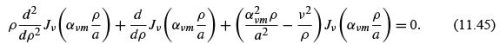Changing the parameter ανm to ανn , we ﬁnd that Jννn ρ/a ) satisﬁes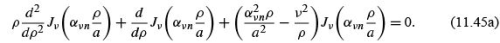Proceeding as in Section 10.2, we multiply Eq. (11.45) by Jννn ρ/a ) and Eq. (11.45a) by Jννm ρ/a ) and subtract, obtaining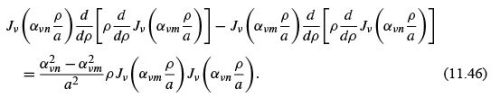Integrating from ρ = 0 to ρ = a , we obtain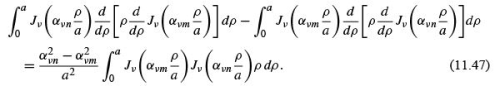Upon integrating by parts, we see that the left-hand side of Eq. (11.47) becomes F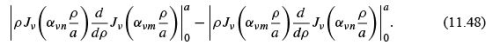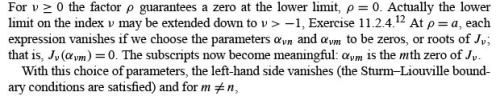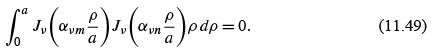This gives us orthogonality over the interval [0,a ].

Normalization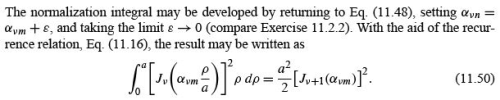Bessel Series

If we assume that the set of Bessel functions Jννm ρ /a ))(ν ﬁxed, m = 1, 2, 3,...)is complete, then any well-behaved but otherwise arbitrary function f(ρ ) may be expanded in a Bessel series (Bessel–Fourier or Fourier–Bessel)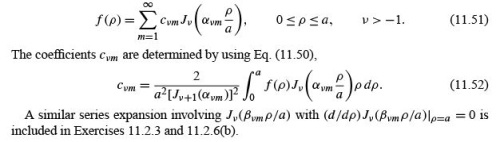Example 11.2.1 ELECTROSTATIC POTENTIAL IN A HOLLOW CYLINDER

From Table 9.3 of Section 9.3 (with α replaced by k ), our solution of Laplace’s equation in circular cylindrical coordinates is a linear combination of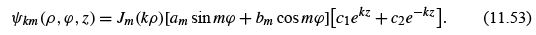The particular linear combination is determined by the boundary conditions to be satisﬁed.
Our cylinder here has a radius a and a height l . The top end section has a potential distribution ψ(ρ, ϕ ). Elsewhere on the surface the potential is zero. The problem is to ﬁnd the electrostatic potential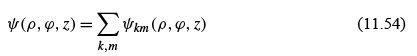everywhere in the interior.
For convenience, the circular cylindrical coordinates are placed as shown in Fig. 11.3.
Since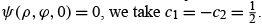The z dependence becomes sinh kz, vanishing at z = 0. The requirement that ψ = 0 on the cylindrical sides is met by requiring the separation constant k to be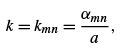where the ﬁrst subscript, m, gives the index of the Bessel function, whereas the second subscript identiﬁes the particular zero of Jm .
The electrostatic potential becomes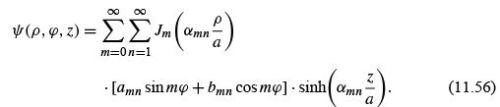Equation (11.56) is a double series: a Bessel series in ρ and a Fourier series in ϕ .
At z = l, ψ = ψ(ρ, ϕ ), a known function of ρ and ϕ . Therefore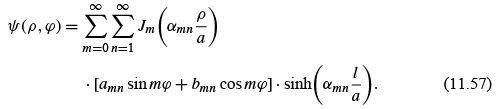The constants amn and bmn are evaluated by using Eqs. (11.49) and (11.50) and the corresponding equations for sin ϕ and cos ϕ (Example 10.2.1 and Eqs. (14.2), (14.3), (14.15) to (14.17)). We ﬁnd 14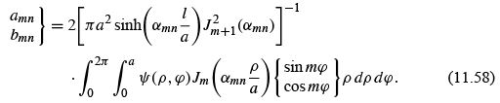These are deﬁnite integrals, that is, numbers. Substituting back into Eq. (11.56), the series is speciﬁed and the potential ψ(ρ, ϕ , z) is determined.

Continuum Form

The Bessel series, Eq. (11.51)  apply to expansions over the ﬁnite interval [0,a ].If a →∞, then the series forms may be expected to go over into integrals.
The discrete roots ανm become a continuous variable α . A similar situation is encountered in the Fourier series,
For operations with a continuum of Bessel functions, Jν (αρ ), a key relation is the Bessel function closure equation,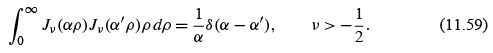This may be proved by the use of Hankel transforms.  An alternate approach, starting from a relation similar to Eq. (10.82), is given by Morse and Feshbach.
A second kind of orthogonality (varying the index) is developed for spherical Bessel functions.

NEUMANN FUNCTIONS,BESSEL FUNCTIONS OF THE SECOND KIND

From the theory of ODEs it is known that Bessel’s equation has two independent solutions.
Indeed, for nonintegral order ν we have already found two solutions and labeled them Jν (x ) and J−ν (x ), using the inﬁnite series (Eq. (11.5)). The trouble is that when ν is integral, Eq. (11.8) holds and we have but one independent solution. A second solution may be developed by the methods of Section 9.6. This yields a perfectly good second solution of Bessel’s equation but is not the standard form.

Deﬁnition and Series Form

As an alternate approach, we take the particular linear combination of Jν (x ) and J−ν (x )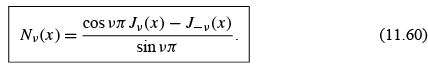This is the Neumann function (Fig. 11.5).15 For nonintegral ν, Nν (x ) clearly satisﬁes Bessel’s equation, for it is a linear combination of known solutions Jν (x ) and J−ν (x ).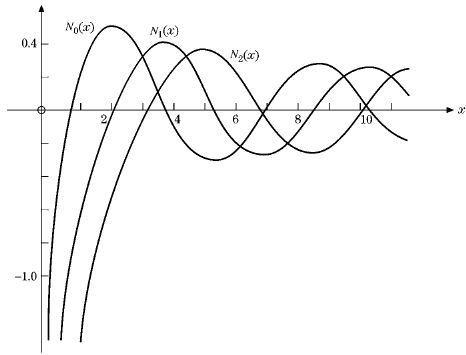FIGURE 11.5 Neumann functions N0 (x ), N1 (x ), and N2 (x ).

Substituting the power-series Eq. (11.6) for n → ν (given in Exercise 11.1.7) yields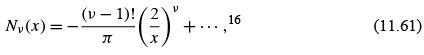for ν> 0. However, for integral ν, ν = n, Eq. (11.8) applies and Eq. (11.60) becomes indeterminate. The deﬁnition of Nν (x ) was chosen deliberately for this indeterminate property. Again substituting the power series and evaluating Nν (x ) for ν → 0 by l’Hôpital’s rule for indeterminate forms, we obtain the limiting value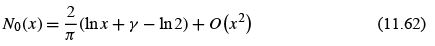for n = 0 and x → 0 , using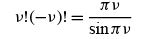(11.63)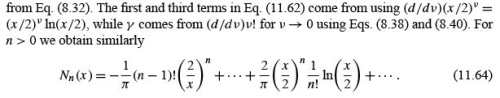Equations (11.62) and (11.64) exhibit the logarithmic dependence that was to be expected.
This, of course, veriﬁes the independence of Jn and Nn .

Other Forms

As with all the other Bessel functions, Nν (x ) has integral representations. For N0 (x ) we have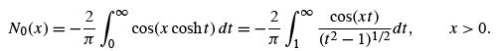These forms can be derived as the imaginary part of the Hankel representations of Exercise 11.4.7. The latter form is a Fourier cosine transform.
To verify that Nν (x ), our Neumann function (Fig. 11.5) or Bessel function of the second kind, actually does satisfy Bessel’s equation for integral n, we may proceed as follows.
L’Hôpital’s rule applied to Eq. (11.60) yields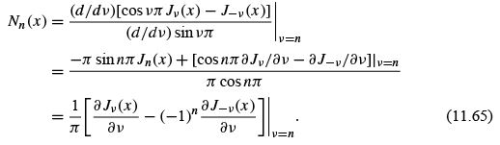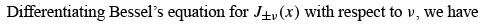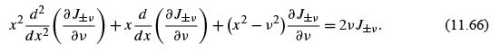Multiplying the equation for J−ν by (−1)ν , subtracting from the equation for Jν (as suggested by Eq. (11.65)), and taking the limit ν → n, we obtain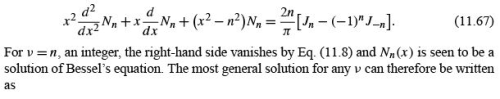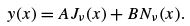(11.68)

It is seen from Eqs. (11.62) and (11.64) that Nn diverges, at least logarithmically. Any boundary condition that requires the solution to be ﬁnite at the origin (as in our vibrating circular membrane (Section 11.1)) automatically excludes Nn (x ). Conversely, in the absence of such a requirement, Nn (x ) must be considered.
To a certain extent the deﬁnition of the Neumann function Nn (x ) is arbitrary. Equations (11.62) and (11.64) contain terms of the form an Jn (x ). Clearly, any ﬁnite value of the constant an would still give us a second solution of Bessel’s equation. Why should an have the particular value implicit in Eqs. (11.62) and (11.64)? The answer involves the asymptotic dependence developed in Section 11.6. If Jn corresponds to a cosine wave, then Nn corresponds to a sine wave. This simple and convenient asymptotic phase relationship is a consequence of the particular admixture of Jin Nn .

Recurrence Relations

Substituting Eq. (11.60) for Nν (x ) (nonintegral ν ) into the recurrence relations (Eqs. (11.10) and (11.12) for Jn (x ), we see immediately that Nν (x ) satisﬁes these same recurrence relations. This actually constitutes another proof that Nν is a solution. Note that the converse is not necessarily true. All solutions need not satisfy the same recurrence relations.

Wronskian Formulas

From Section 9.6 and Exercise 10.1.4 we have the Wronskian formula17 for solutions of the Bessel equation,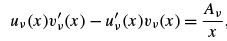(11.69)

in which Aν is a parameter that depends on the particular Bessel functions uν (x ) and vν (x ) being considered. Aν is a constant in the sense that it is independent of x . Consider the special case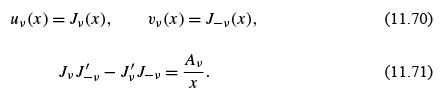Since Aν is a constant, it may be identiﬁed at any convenient point, such as x = 0. Using the ﬁrst terms in the series expansions (Eqs. (11.5) and (11.6)), we obtain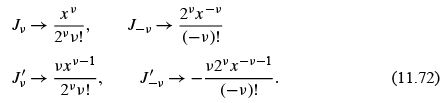Substitution into Eq. (11.69) yields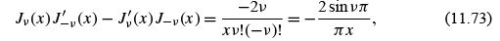using Eq. (8.32). Note that Aν vanishes for integral ν , as it must, since the nonvanishing of the Wronskian is a test of the independence of the two solutions. By Eq. (11.73), Jand J−n are clearly linearly dependent.
Using our recurrence relations, we may readily develop a large number of alternate forms, among which are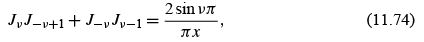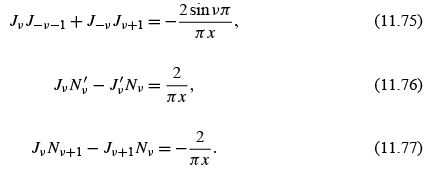Many more will be found in the references given at chapter’s end.
Wronskians were of great value in two respects: (1) in establishing the linear independence or linear dependence of solutions of differential equations and (2) in developing an integral form of a second solution. Here the speciﬁc forms of the Wronskians and Wronskian-derived combinations of Bessel functions are useful primarily to illustrate the general behavior of the various Bessel functions. Wronskians are of great use in checking tables of Bessel functions.

Example 11.3.1 COAXIAL WAVE GUIDES

We are interested in an electromagnetic wave conﬁned between the concentric, conducting cylindrical surfaces ρ = a and ρ = b. To go from the standing wave of these examples to the traveling wave here, we let A = iB, A = amn ,B = bmn in Eq. (11.40a) and obtain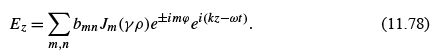For the coaxial wave guide one generalization is needed. The origin, ρ = 0, is now excluded (0 <a ≤ ρ ≤ b). Hence the Neumann function Nm (γ ρ ) may not be excluded. Ez (ρ ,ϕ ,z,t ) becomes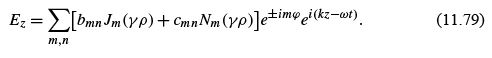With the condition

Hz = 0,                        (11.80)

we have the basic equations for a TM (transverse magnetic) wave.

The (tangential) electric ﬁeld must vanish at the conducting surfaces (Dirichlet boundary condition), or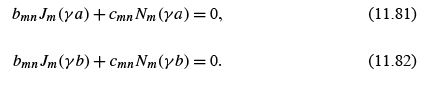These transcendental equations may be solved for γ(γmn ) and the ratio cmn /bmn .From Example 11.1.2,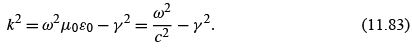Since k2 must be positive for a real wave, the minimum frequency that will be propagated (in this TM mode) is

ω = γc,         (11.84)

with γ ﬁxed by the boundary conditions, Eqs. (11.81) and (11.82). This is the cutoff frequency of the wave guide.
There is also a TE (transverse electric) mode, with Ez = 0 and Hz given by Eq. (11.79).
Then we have Neumann boundary conditions in place of Eqs. (11.81) and (11.82). Finally, for the coaxial guide (not for the plain cylindrical guide, a = 0), a TEM (transverse electromagnetic) mode, Ez = Hz = 0, is possible. This corresponds to a plane wave, as in free space.
To conclude this discussion of Neumann functions, we introduce the Neumann function Nν (x ) for the following reasons:

1. It is a second, independent solution of Bessel’s equation, which completes the general solution.

2. It is required for speciﬁc physical problems such as electromagnetic waves in coaxial cables and quantum mechanical scattering theory.

3. It leads to a Green’s function for the Bessel equation.
4. It leads directly to the two Hankel functions .

159 docs

,

,

,

,

,

,

,

,

,

,

,

,

,

,

,

,

,

,

,

,

,

,

,

,

;